Function Repository Resource:

# GraphElementData

Get information about named functions used in VertexShapeFunction and EdgeShapeFunction

Contributed by: Jaebum Jung
 ResourceFunction["GraphElementData"]["shape"] represents a function that generates a shape for vertices or edges when applied to VertexShapeFunction or EdgeShapeFunction in a graph. ResourceFunction["GraphElementData"]["shape","property"] gives the specified property for the named function "shape". ResourceFunction["GraphElementData"][{"shape",opts},"property"] gives the specified property for the named function "shape" with the options opts.

## Details

ResourceFunction["GraphElementData"] is typically used as a setting for VertexShapeFunction and EdgeShapeFunction in different graph functions.
ResourceFunction["GraphElementData"]["shape"]["coords","element","metadata"] gives the graphics primitives used to render a vertex or edge in a graph.
Possible properties in ResourceFunction["GraphElementData"]["shape","property"] include "Preview", "Options" and "ElementPreview".
VertexShapeFunctionResourceFunction["GraphElementData"]["shape"] specifies that each vertex should be rendered with the primitives provided by ResourceFunction["GraphElementData"]["shape"][{x,y},v,{w,h}], where {x,y} is the center, v is the vertex name, and {w,h} are the width and the height.
EdgeShapeFunctionResourceFunction["GraphElementData"]["shape"] specifies that each edge should be rendered with the primitives provided by ResourceFunction["GraphElementData"]["shape"][{{x1,y1},{x2,y2},},vw], where {{x1,y1},{x2,y2},} are line segments and vw is the edge.
The data resource VertexShapeFunction contains all named vertex functions. Use ResourceData["VertexShapeFunction"] to get a list of them.
The data resource EdgeShapeFunction contains all named vertex functions. Use ResourceData["EdgeShapeFunction"] to get a list of them.

## Examples

### Basic Examples

Preview the "HalfFilledArrow" edge shape function:

 In:=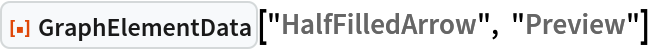Out=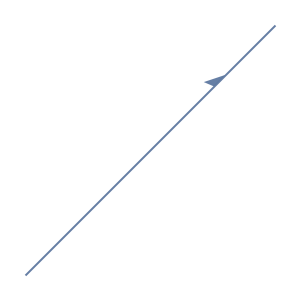List all supported options of the "HalfFilledArrow" edge shape function:

 In:=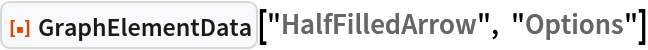Out=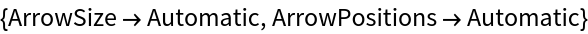Draw edges using the "HalfFilledArrow" edge shape function:

 In:=Out=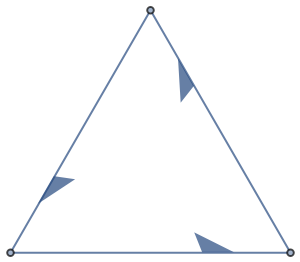Preview the "Square" vertex shape function:

 In:=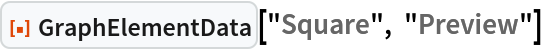Out=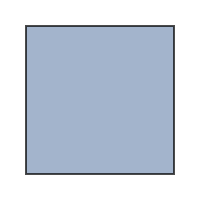Draw vertices using the "Square" vertex shape function:

 In:=Out=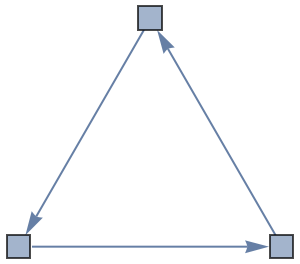## Version History

• 1.0.0 – 20 March 2023